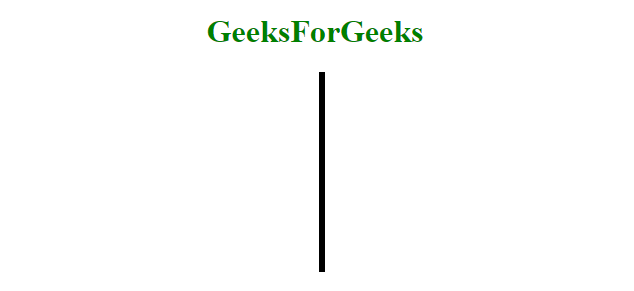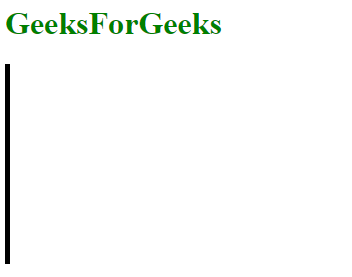# How to make a vertical line using HTML ?

• Difficulty Level : Medium
• Last Updated : 10 May, 2022

To make a vertical line, use border-left or border-right property. The height property is used to set the height of border (vertical line) element. Position property is used to set the position of vertical line.

Example 1: It creates a vertical line using border-left, height and position property.

## html

 ```<``html``>` `<``head``>``    ``<``title``>``        ``HTML border Property``    ````    ` `    ````    ``<``style``>``        ``.vertical {``            ``border-left: 6px solid black;``            ``height: 200px;``            ``position:absolute;``            ``left: 50%;``        ``}``    ````` `<``body` `style` `= ``"text-align: center;"``>` `    ``<``h1` `style` `= ``"color: green;"``>``        ``GeeksForGeeks``    ````        ` `    ``<``div` `class` `= ``"vertical"``>` `` `                                     `

Output:Example 2: It creates a vertical line using border-left and height property.

## html

 ```<``html``>` `<``head``>``    ``<``title``>``        ``HTML border Property``    ````    ` `    ````    ``<``style``>``        ``.vertical {``            ``border-left: 5px solid black;``            ``height: 200px;``        ``}``    ````` `<``body``>` `    ``<``h1` `style``= ``"color: green;"``>``        ``GeeksForGeeks``    ````        ` `    ``<``div` `class``= ``"vertical"``>` `` `                   `

Output:Supported Browsers: